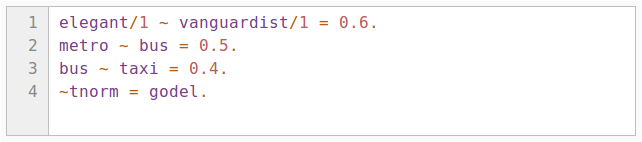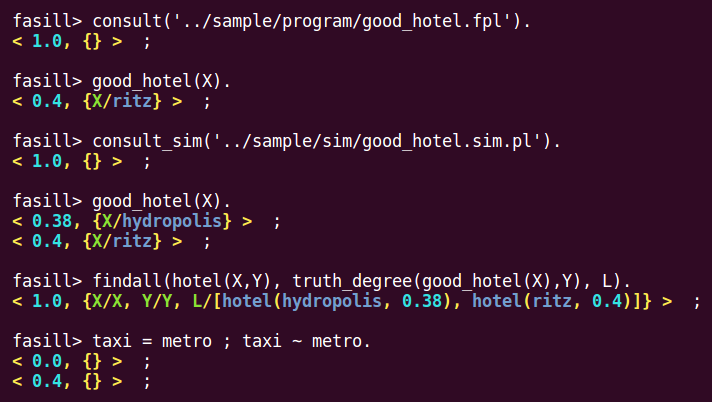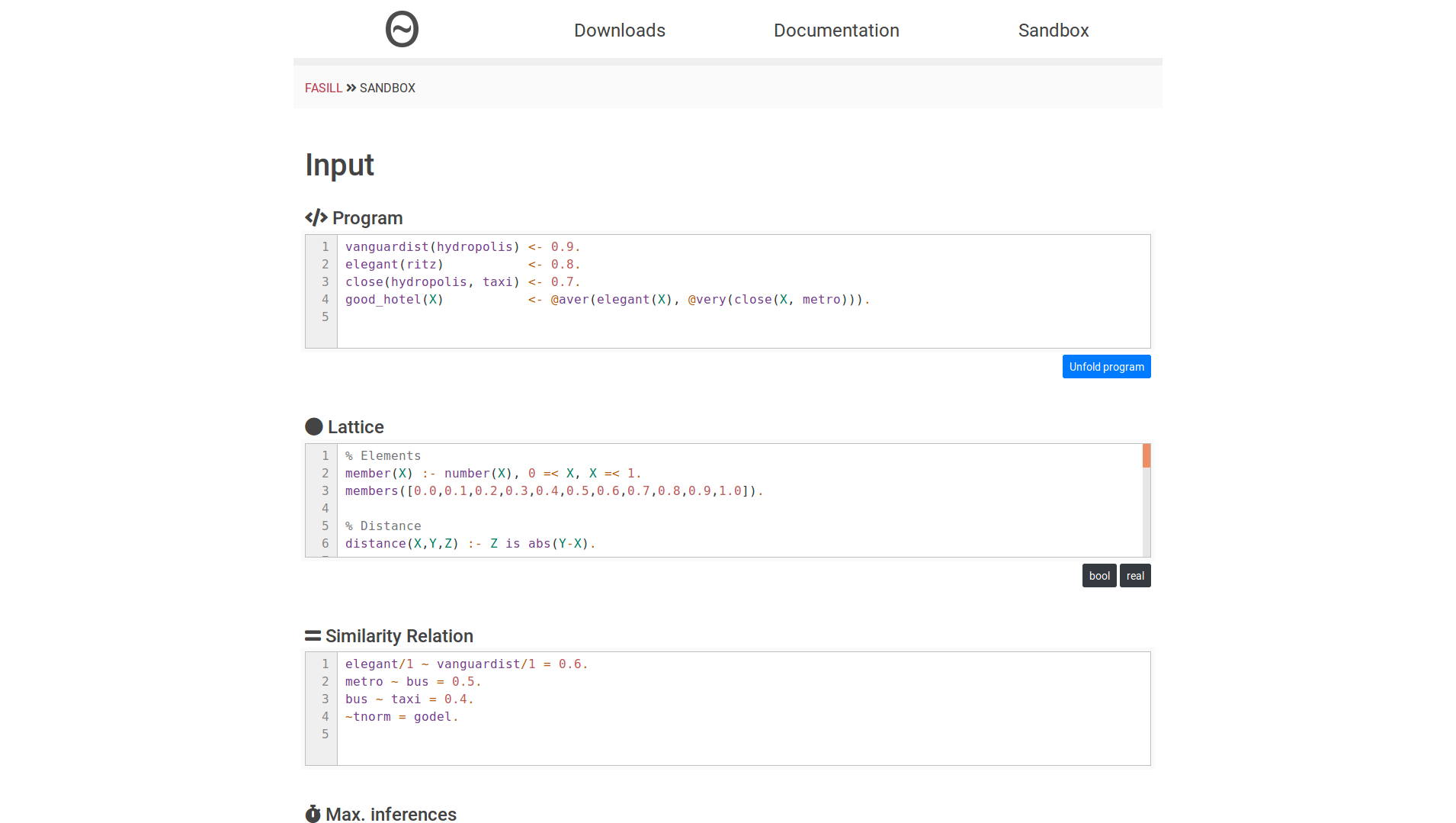### What is FASILL?

FASILL is a Prolog-like first order language containing variables, function symbols, predicate symbols and constants. To increase language expressiveness, FASILL also contains the truth values of a complete lattice, equipped with a collection of connectives to combine/propagate truth values through the rules.

#### Rules

A FASILL program is a tuple <Π, R, L> where Π is a set of rules, R is a similarity relation between the elements of the signature Σ of Π, and L is a complete lattice. A rule has the form A ← B, where A is an atomic formula called head and B, called body, is a well-formed formula (built from atomic formulas B1, ..., Bn, truth values of the lattice and connectives).

#### Complete Lattices

All relevant components of each lattice can be encapsulated inside a Prolog file which must contain the definitions of a minimal set of predicates defining the set of valid elements, the full or partial ordering established among them, as well as the repertoire of fuzzy connectives which can be used for their subsequent manipulation.

#### Similarity Relations

Given a domain U and a lattice L with a fixed t-norm, a similarity relation R is a fuzzy binary relation on U fulfilling the reflexive, symmetric and transitive properties. FASILL takes a set of similarity equations f/n ~ g/n = r, where f and g are propositional variables or constants and r is an element of L, and generates a valid similarity relation by applying the reflexive, symmetric and transitive closures over the initial scheme.#### Built-in Predicates

FASILL has a large set of built-in predicates for arithmetic comparison, arithmetic evaluation, atom processing, control constructs, term comparison, term unification, type testing, list manipulation, etc.

### Weak Unification and Operational Semantics

As a logic language, FASILL inherits the concepts of substitution, unifier and most general unifier. Some of them are extended to cope with similarities. Concretely, the most general unifier is replaced by the concept of weak most general unifier and a weak unification algorithm is introduced to compute it.

The procedural semantics of FASILL is defined in a stepwise manner. First, an operational stage is introduced which proceeds similarly to SLD resolution in pure logic programming, returning an expression still containing values and connectives. Then, an interpretive stage evaluates these connectives and produces a final answer.##### Interactive console

The FASILL interactive console provides programmers with a quick way to execute fuzzy logic goals.##### Online sandbox

You can try FASILL out right now, without installing anything. If you like it, you can install it.